# Circular Motion AP Physics C Mrs Coyle Circular

• Slides: 15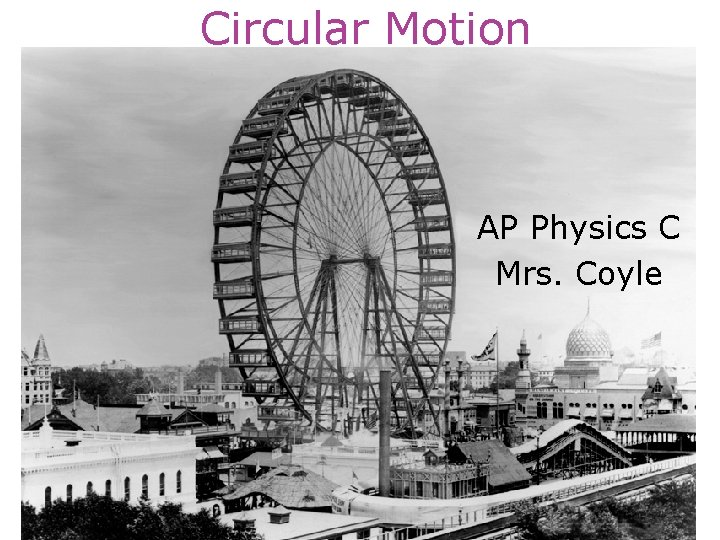Circular Motion AP Physics C Mrs. CoyleCircular Motion • Uniform circular motion (constant centripetal acceleration) • Motion with a tangential and radial (centripetal)acceleration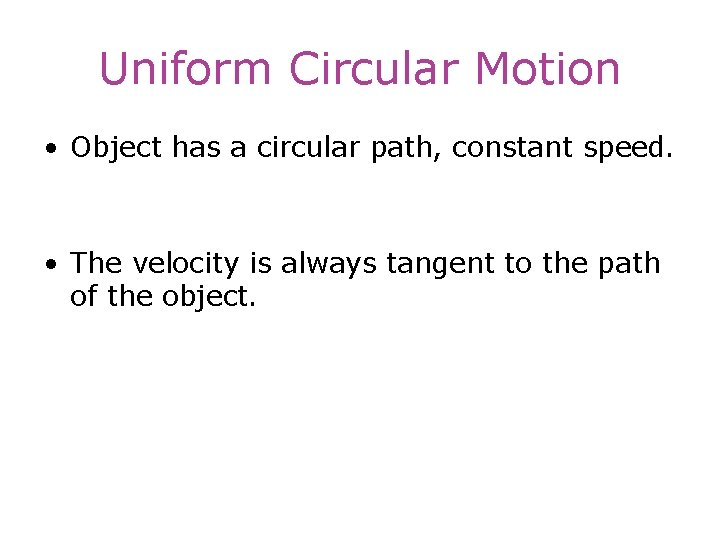Uniform Circular Motion • Object has a circular path, constant speed. • The velocity is always tangent to the path of the object.Why is there acceleration in uniform circular motion? Dv = vf – vi a= Dv/ Dt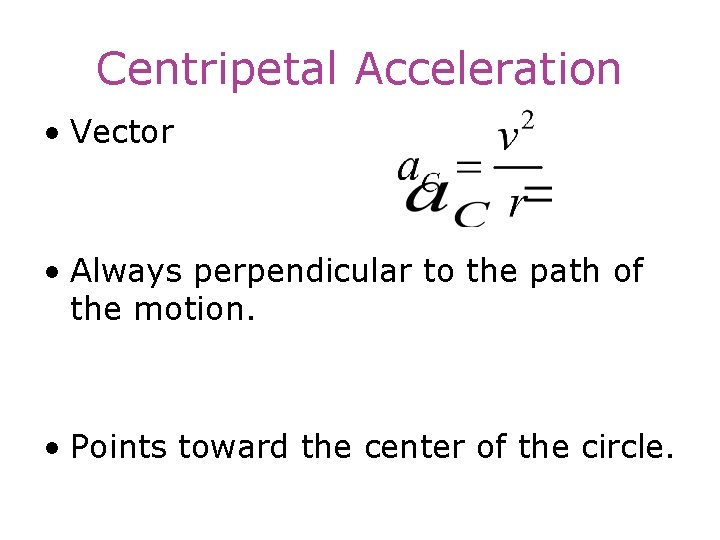Centripetal Acceleration • Vector • Always perpendicular to the path of the motion. • Points toward the center of the circle.Characteristics of Uniform. Circular Motion • Tangential (linear) Velocity • Frequency • Period • Centripetal Acceleration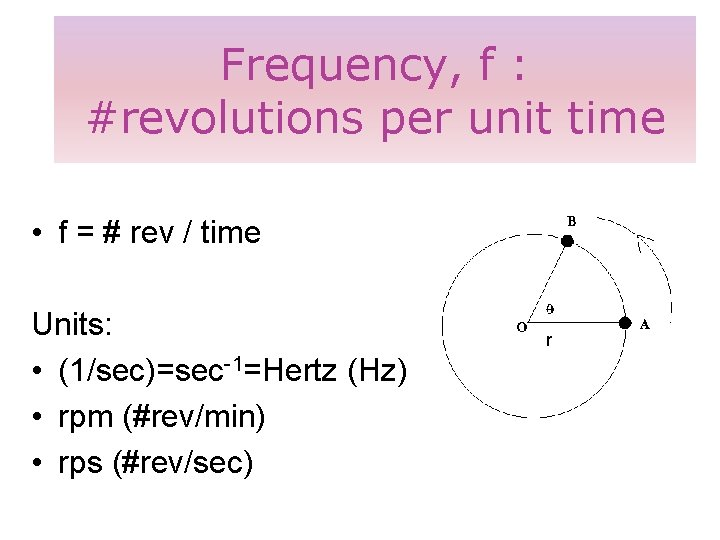Frequency, f : #revolutions per unit time • f = # rev / time Units: • (1/sec)=sec-1=Hertz (Hz) • rpm (#rev/min) • rps (#rev/sec) rPeriod • Period T : time for 1 revolution – Unit: sec, min, h • Relating Frequency and period f= 1 T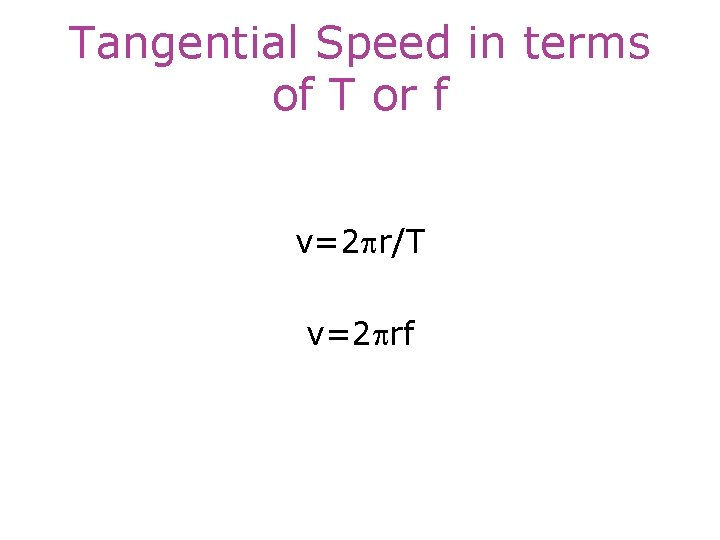Tangential Speed in terms of T or f v=2 pr/T v=2 prfExample 1 • A boy whirls a stone in a horizontal circle of r=1. 5 m, 2 m above the ground. The string breaks and the stone strikes 10 m away. What was the centripetal acceleration during the circular motion? • Answer: 160 m/s 2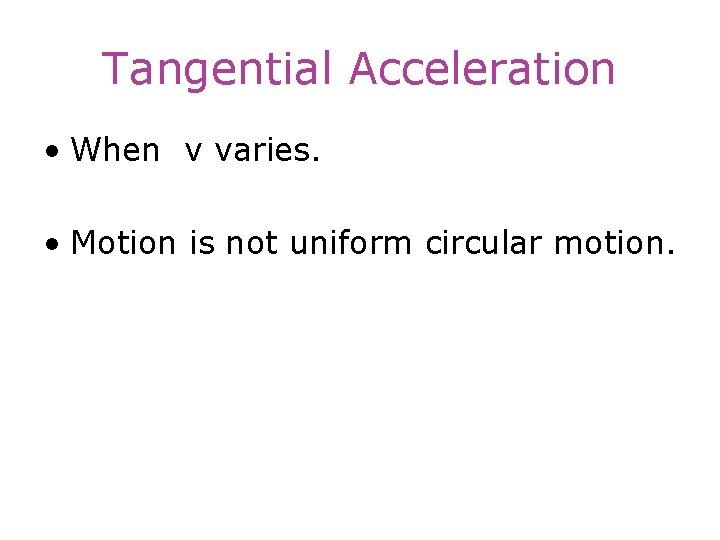Tangential Acceleration • When v varies. • Motion is not uniform circular motion.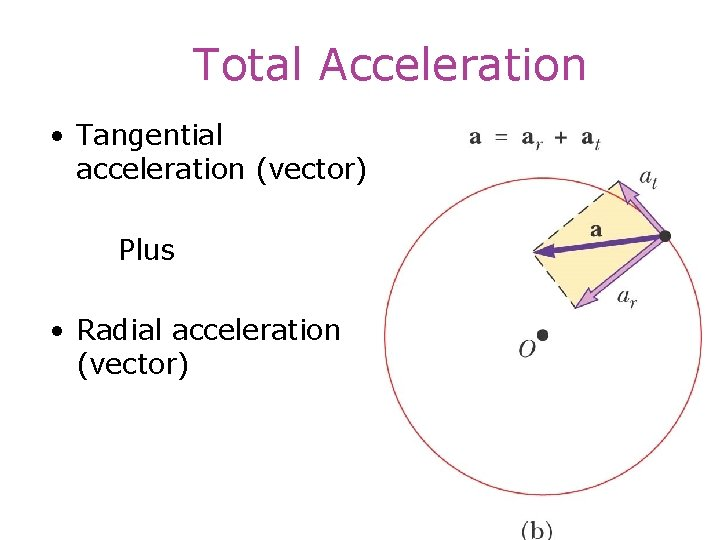Total Acceleration • Tangential acceleration (vector) Plus • Radial acceleration (vector)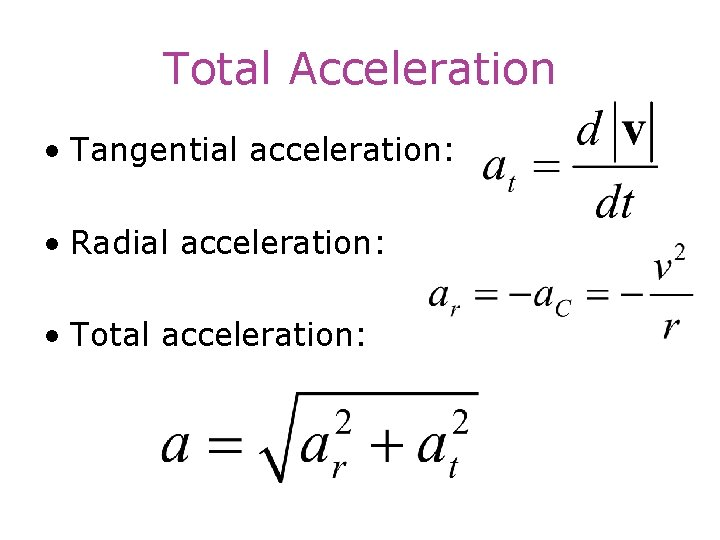Total Acceleration • Tangential acceleration: • Radial acceleration: • Total acceleration: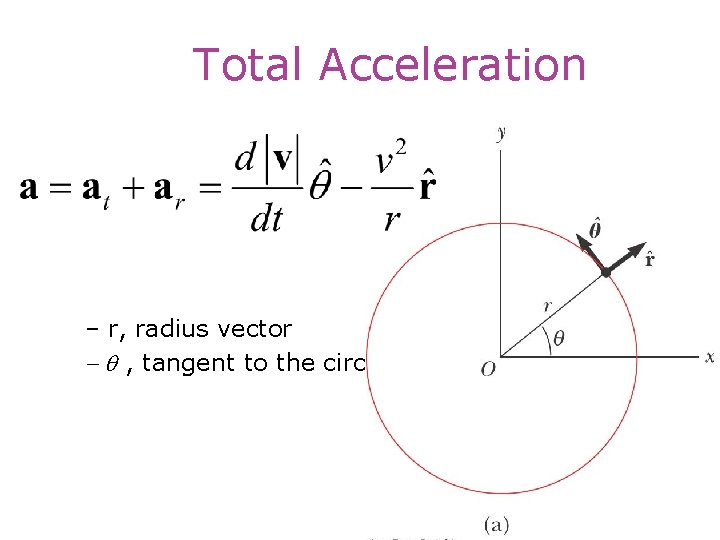Total Acceleration – r, radius vector - q , tangent to the circle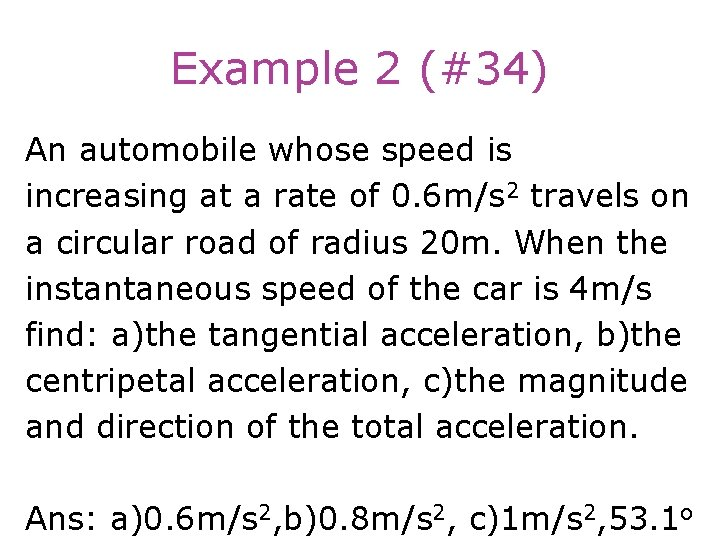Example 2 (#34) An automobile whose speed is increasing at a rate of 0. 6 m/s 2 travels on a circular road of radius 20 m. When the instantaneous speed of the car is 4 m/s find: a)the tangential acceleration, b)the centripetal acceleration, c)the magnitude and direction of the total acceleration. Ans: a)0. 6 m/s 2, b)0. 8 m/s 2, c)1 m/s 2, 53. 1 o